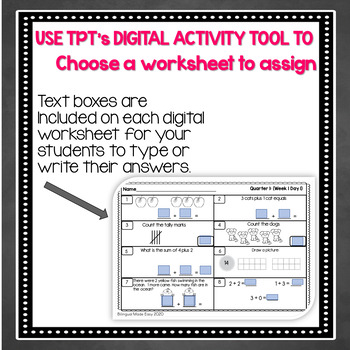# First Grade Math Morning Work FREEBIE in English & Spanish1st
Subjects
Standards
Resource Type
Formats Included
• PDF
•Activity
Pages
10 pages
Easel Activity Included
This resource includes a ready-to-use interactive activity students can complete on any device. Easel by TpT is free to use! Learn more.

### Description

USE AS A DIGITAL PRODUCT or PRINT THE PDF

Help your English Language Learners master the first 8 weeks of the 1st grade Common Core Math curriculum. These bilingual (English & Spanish) worksheets are a spiral review of the skills taught in Kindergarten as well as the skills taught in the beginning of first grade. They are perfect for morning work or can be sent home for homework. Each worksheet in English has a duplicate copy in Spanish. These worksheets can be printed double sided in order to accommodate all of the students in class. I absolutely LOVE this product! I whole heartedly believe in constantly reviewing, practicing, and previewing concepts throughout the year in addition to focusing on one specific topic at a time with investigative activities where students can build their conceptual understanding of the topic. I cannot think of a better way to constantly review and practice the 1st grade math objectives in a time-efficient way than this.

Total Pages
10 pages
N/A
Teaching Duration
N/A
Report this Resource to TpT
Reported resources will be reviewed by our team. Report this resource to let us know if this resource violates TpT’s content guidelines.

### Standards

to see state-specific standards (only available in the US).
Determine the unknown whole number in an addition or subtraction equation relating three whole numbers. For example, determine the unknown number that makes the equation true in each of the equations 8 + ? = 11, 5 = ▯ - 3, 6 + 6 = ▯.
Add and subtract within 20, demonstrating fluency for addition and subtraction within 10. Use strategies such as counting on; making ten (e.g., 8 + 6 = 8 + 2 + 4 = 10 + 4 = 14); decomposing a number leading to a ten (e.g., 13 - 4 = 13 - 3 - 1 = 10 - 1 = 9); using the relationship between addition and subtraction (e.g., knowing that 8 + 4 = 12, one knows 12 - 8 = 4); and creating equivalent but easier or known sums (e.g., adding 6 + 7 by creating the known equivalent 6 + 6 + 1 = 12 + 1 = 13).
Relate counting to addition and subtraction (e.g., by counting on 2 to add 2).
Solve word problems that call for addition of three whole numbers whose sum is less than or equal to 20, e.g., by using objects, drawings, and equations with a symbol for the unknown number to represent the problem.
Use addition and subtraction within 20 to solve word problems involving situations of adding to, taking from, putting together, taking apart, and comparing, with unknowns in all positions, e.g., by using objects, drawings, and equations with a symbol for the unknown number to represent the problem.